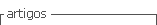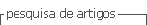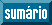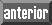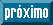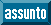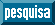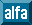## Artigo

•Citado por SciELO
•Acessos

•Citado por Google
•Similares em SciELO
•Similares em Google

## versão impressa ISSN 0122-5383versão On-line ISSN 2382-4581

### C.T.F Cienc. Tecnol. Futuro vol.9 no.1 Bucaramanga jan./jun. 2019

#### http://dx.doi.org/10.29047/01225383.147

Artículos originales

METHODOLOGY TO DEFINE HYDROCARBON POTENTIAL IN A SHALE RESERVOIR BASED ON GEOCHEMICAL DATA AND WELL LOGS

METODOLOGÍA PARA DEFINIR EL POTENCIAL DE HIDROCARBUROS EN UN SHALE PLAY A PARTIR DE DATOS DE GEOQUÍMICA Y REGISTROS DE POZOS

Gutiérrez-Torres, Ludy-Amparoa  *  , Molina-Gomez, Luz-Dianaa  , Ribón-Barrios, Helena-Margaritaa  , Aristóbulo Bejaranob  , Tatiana-Milena Juliao-Lemusc

a Universidad Industrial de Santander, carrera 27 calle 9, C.P 680002, Bucaramanga, Colombia.

b SPS-oil Ltda, Bucaramanga, Santander, Colombia.

c Ecopetrol- Instituto Colombiano del Petróleo, km 7 vía a Piedecuesta - Bucaramanga, C.P 681011, Piedecuesta, Colombia.

ABSTRACT

The office U.S. Energy Information Administration (EIA) has suggested significant volumes of hydrocarbon resources in unconventional Shale type reservoirs, which happens to be very interesting nowadays.

The complexity of these reservoirs, along with the high level of risk during the exploration stage, and the lack of laboratory data, are challenging for an adequate estimation of hydrocarbon volumes in shale reservoirs. An innovative methodology to estimate prospective resources on a Shale reservoir is proposed in this paper, based on petrophysical and geochemical data from well logs, such as porosity, hydrocarbon saturation, TOC (total organic content), gas content, thermal rock maturity, clay fraction, thickness, rock density, etc, all of them using Monte Carlo simulation.

Further, this paper proposes a new way of interpreting petrophysical data to obtain a clearer view of reservoir characterization, especially Brittleness, which is of great relevance to define the potential of fracturing and hydrocarbon production. The methodology was applied to the Tablazo Formation in the Middle Magdalena Valley Basin (MMVB) in Colombia. The results show a total best estimate of oil in place (OOIP) of 51 637 Bls/acre, gas adsorbed in place 39.72 Mcf/acre, and free gas in place of 177.18 Mcf/acre. Comparing these results with those obtained by applying other methodologies, the best estimates of oil in place is 146 933 Bls/ acre, gas adsorbed in situ 40.57 Mcf/acre, and free gas in place of 504.07 Mcf/acre. Data reported in the literature, on the same area, corroborate these results.

To conclude, with this methodology a new approach is achieved for estimating prospective resources in Shale reservoirs with better results using the Monte Carlo simulation.

Key words: Shale; Resources; Montecarlo; Geochemistry; Petrophysics

RESUMEN

La complejidad de los yacimientos no convencionales tipo Shale, aunada con el alto nivel de riesgo en etapas exploratorias y la escasa información de Laboratorio, plantea un desafío para la adecuada estimación de los volúmenes de hidrocarburo que los yacimientos shale pueden ofrecer.

Este trabajo propone una metodología innovadora para estimar los recursos prospectivos en un yacimiento tipo shale a partir de datos petrofísicos y geoquímicos extraídos de registros de pozo, tales como porosidad, saturación de hidrocarburos, TOC (contenido orgánico total), Contenido de Gas, madurez termal de la roca, fracción de arcilla, espesor, densidad de la roca, entre otros, aplicando simulación Monte Carlo.

Adicionalmente, se plantea una nueva forma de interpretar los datos petrofísicos para obtener una visión más clara de la caracterización del yacimiento, especialmente del Brittleness que es de gran importancia para definir el potencial de fracturamiento y por lo tanto de producción que tiene la formación. La metodología se aplicó a la formación Tablazo en la Cuenca del Valle Medio del Magdalena (VMM - Colombia). Los resultados de mejor estimado arrojan un aceite total en sitio (OOIP) de 51 637 Bls/acre, gas adsorbido en sitio de 39.72 Mcf/acre y gas libre en sitio de 177.18 Mcf/acre. Al comparar estos resultados con los obtenidos al aplicar otras metodologías y datos reportados en la literatura sobre la misma zona, corroboran los resultados obtenidos.

En conclusión, con esta metodología, se logra un nuevo enfoque de estimación de recursos prospectivos en yacimientos Shale con resultados más ajustados al usar simulación Montecarlo.

Palabras-clave: Shale; Recursos; Montecarlo; Geoquímica; Petrofísica

1. INTRODUCTION

In shale reservoirs, the gas in place is stored as free gas in the pores and fractures and it is adsorbed gas in the matrix. Therefore, the storage properties and flow properties, the thermal maturity and quality of the rock, should be defined.

During the exploration phase of shale reservoirs, due to the high associated commercial risk, the available data is originated in well logs, opposed to what happens with laboratory data. Hence, the log analysis to be performed is not the conventional one. Therefore, it is necessary to have proper interpretation skills for this type of reservoir to determine the geochemical and petrophysical properties of the rock. This will reduce the uncertainty associated with pertinent estimations.

The document presents a methodology to define hydrocarbon potential in the discovery stage of shale reservoirs. This is related to the innovative interpretation of the well logs using probabilistic simulation.

2. THEORETICAL FRAME

The basis for carrying out the right hydrocarbon potential estimation in a shale reservoir is an adequate reservoir characterization. The challenge is the definition of a complex and heterogeneous reservoir in terms of petrophysical, geochemical and fluid properties. The Shale formation is composed not only by clay minerals but, it also contains large volumes of quartz, carbonates and other minerals. Therefore, it is very useful to evaluate reservoir quality and, furthermore, to define the hydraulic fluid units to thus obtain deeper and clearer understanding of the reservoir. Based on the mineralogical composition, we can estimate the brittleness index, which is an important factor to predict fracture parameters to produce a gas shale play.

On the other hand, the organic richness and the thermal maturity are highly relevant parameters in shale reservoirs. The total organic content (TOC) represents the organic material quality, while the thermal maturity related with temperature is the gradual decrease of the kerogen content until producing hydrocarbon expulsion and a change in the TOC. The fact that a reservoir has organic material does not imply that hydrocarbon generation has occurred. The generation depends on the thermal maturity level; in fact, the rock maybe has a high TOC, but could not be a source rock.

METHODOLOGY TO QUANTIFY PROSPECTIVE HYDROCARBON RESOURCES IN SHALES

In accordance with the Petroleum Resources Management System (PRMS) in early and exploration stages in high risk ventures - such as in shale plays - the quantifying method used to estimate resources is the volumetric method. According to Crain, 2015  for a shale reservoir, the initial gas in place is the sum of the free gas and the adsorbed gas.

Where:

A = Area, acres

Bg = Gas formation volumetric factor, cf/scf

GIPtotal = Total gas in-place, MCF

H = Thickness, ft

K1 = Units conversion factor; 43560*10A-6

OGIPfree = Original free gas in place, Mcf

PHIt = Total porosity, fraction

PHIe = Effective porosity, fraction

Swt = Total water saturation, fraction

Swe = Effective water saturation, fraction

Where:

A = Area, acres

D = Layer density from log or lab measurement, P b (g/cm3)

GC = Gas content, scf/ton

H = Thickness, ft

KG6 = units conversion factor; 1.3597x10-3

The typical shale densities are in the range of 2.20 to 2.60 g/cm3. 

For oil reservoirs:

Where:

Bo = Oil formation volumetric factor, Bls/STB

K2 = Units conversion factor; 7758

OOIP = Original total oil in place, STB

To determine the parameters of effective porosity and water saturation, the Crain method  uses a porosity corrected for Kerogen and Shale. That is, the volume of shale, the fraction by weight of the TOC, and the volume fraction of Kerogen must be determined and then the porosity corrected. The water saturation is determined by means of the Schlumberger dual water saturation equation as reference. The proposed methodology incorporates new correlations proposed by Aristóbulo Bejarano (verbal communication, 2014) for effective porosity and water saturation.

Parameters such as the weight fraction of TOC, gas content and thickness are determined as proposed by the Crain method

According to Crain , the gas content can be obtained using the Langmuir adsorption curve, which can be derived from reservoir temperature and pressure. The correlation of Gc (Gas Content) with TOC described below seems adequate, but the Langmuir method would be a useful calibration step.

This methodology is not taken into account because the respective data are not available. Figure 1 shows a scheme of the proposed methodology to obtain data involving the volumetric equation.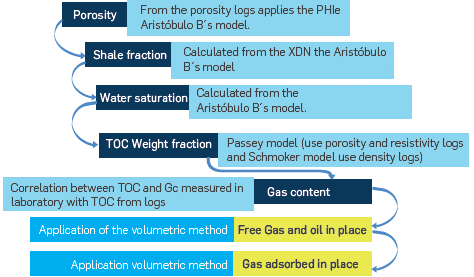Figure 1 Procedure developed to calculate critical parameters such as porosity, water saturation and organic material content (TOC%) to apply the required volumetric method equations.

The methodology applies to prospective resources, thus the most appropriate tool is to use a probabilistic simulation to perform the estimation under uncertainty and risk. This defines the probability tendencies for the parameters involved. A simulation was processed using Monte Carlo simulation to obtain the low, better and high estimations for prospective resources in order to evaluate the volumetric method. (Monte Carlo simulation with 1 000 000 iterations) using the Cristal Ball Software 

The Monte Carlo simulation (MCS) in theory is a technique that combines the statistics concepts (aleatory sampling) with the computer capacity to generate pseudo aleatory numbers and automatized calculations. Variables Probability distribution is used The values of the forecast answers from areas or reservoirs being studied could improve the reservoir characterization and allow for defining and quantifying the most probable answers.

3. EXPERIMENTAL DEVELOPMENT

The northern part of South America has formations with shale gas and shale oil potential in Cretaceous marine deposits. The Cretaceous shales are rich in organic material and are similar in age to the Eagle Ford of Texas and Niobrara formations in the United States.

In South America Basins, the Middle Magdalena Valley Basin (MMVB) is one of the most explored Basins in Colombia, mostly in the Paleogene section. A century of exploration history in the MMVB has discovered important gas and oil reserves. According to Vargas , La Luna Formation is the main source rock in the MMVB. The interval corresponding to the Tablazo, Paja and Simití formations is second. According to reports from Sintana Energy in its web page (http://www.sintanaenergy.com/), the Cretaceous section is close to 4 000 to 8 000 feet and it has been recognized as one of the most important source rocks in the world. With the increased interest for unconventional reservoirs, these formations represent a significant exploration potential for shale plays.

The Tablazo formation is composed of organic material mainly of kerogen type II, with a marine environment of half platforms and calcareous shale interbeddings with blue hard limestones.

To evaluate the shale zone in the Tablazo formation, Well logs were used for the case study. The logs are Gamma Ray, Caliper, Resistivity (Induction log, ILD), Neutron porosity and Density. We worked with reported depth data between 10 548 to 10 950 ft, with net pay between 150 to 402 ft, and a huge amount of data in the interest section. As regards geochemistry, data required to apply the methodology proposal, it was based on the information found in literature relative to the Tablazo formation and the Flecha X well study. Table 1, shows data from the study zone.

Table 1 Data reported in the literature concerning Tablazo formation and Flecha X well study.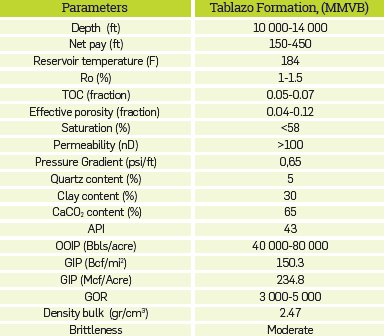Source: US, Energy Information Administration, 2013 and Sintana Energy, 2013.

DETERMINATION OF EFFECTIVE POROSITY.

Effective porosity is calculated using the Bejarano's effective porosity model. (Verbal communication, direct):

Where:

PHID = density log porosity, fraction

PHIN = porosity of the Neutron log, fraction

pma- = matrix density, g/cm3

pf = fluid density, generally it depends of the water density (filtered) and the amount and hydrocarbon type, g/cm3

αL (alfa read) = XDN=slope density-neutron

αSh = min (XDN)=point of saturated clay 100% water, where the slope value is the minimum XDN reading

This methodology allows build an XDN log that can be visualized together with the gamma ray log to define layers of interest.

DETERMINATION OF THE WATER SATURATION.

The water saturation is calculated from the water saturation model of Bejarano A. (verbal communication). This model is based on the rocks behavior rich in organic content. Figure 2 is a scheme of the solids and fluid components in source rocks and non-source rocks. It is assumed that the rocks that are organically rich are composed of three components: (1) the rock matrix, (2) the solid and organic matter, and (3) the saturated fluid(s) in the porous space.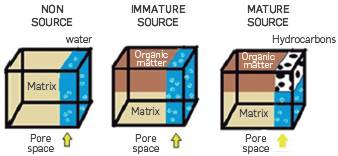Figure 2 Schematization of solid and fluid components in source and non-source rocks.

The equations of Total water saturation from Aristobulo B. are:

The total water saturation is equivalent to Swt = XSH due that XSH resistivity value is related to micro-porosity and this has a water saturation of one (1). The water saturation in porosity where the hydrocarbon content (Swo) is present, the water saturation is zero (0) because in this case the rock is hydrocarbon wet. One of the calculated shale fractions is:

Where:

PHIN = porosity reading of the neutron log, fraction

PHNsh = shale porosity from the Neutron log, fraction

XDN = quotient density-neutron, slope

XSH = shale fraction

αSh = saturated clay 100% water, XDN minimum reading

It must be noted than XSH ≥ Swt and Swt is equivalent to Swi (Irreducible water saturation).

DETERMINATION OF THE GAS CONTENT.

The gas content is calculated from the total organic carbon TOC. The correlation used for the gas content involves TOC from the logs below:

Where:

Gc = gas content, scf/ton

KG11 = range of the gas conversion factor from 5 to 15, default = 9 

TOC% = total organic carbon

A visual analysis of the TOC from logs for the organic content is based on the porosity-resistivity superposition technique, which is widely used to locate possible hydrocarbon indicators in the analysis of conventional logs. The weight fraction of the TOC can then be calculated from the porosity and resistivity logs using the Passey method  or the Schmoker method .

The Passey model  is frequently called the SlogR when based on Sonic logs (transit time). If an adequate transit time curve is not available, the density or neutron curves can be used in the Passey model as shown by .

Where:

DENS = apparent density value, g/cm3

DENSbase= apparent density baseline in non-source, g/cm3

DTC = transit time, sonic log reading in any zone, usec/ft

DTCbase = sonic baseline in non-source rock, usec/ft

LOM = Level of organic maturity, dimensionless

RESD = resistivity, ohm/m

RESDbase = deep resistivity baseline in non-source rock, ohm/m

SFls.d.n = scale factor to calibrate to lab values of TOC

WTOC = total organic carbon, weight fraction

XlogR = Passey's number (separations) of the logs where X is equal to Sonic (s), density (D) or neutron (N) fraction

Y = density log (DENS), sonic log (DT) o neutron log (PHIN)

A = 0.02 for sonic log, 2.50 for density logging and 4.00 for neutron logging

The LOM (Level of Organic Maturity) is rarely measured but it is function of vitrinite reflectance (Ro). According to Charsky , the vitrinite reflectance values (Ro) can be converted to LOM with the graph in Figure 3.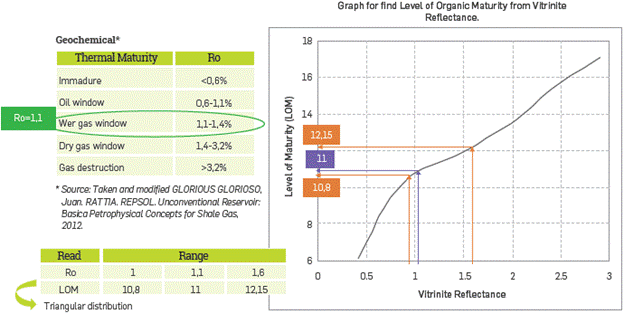Figure 3 Graph to calculated the Level of Organic Maturity from Vitrinite Reflectance. Calculating the Level of thermal maturity Tablazo Formation, (MMVB). Based on CRAIN. 

The Figure 4 shows the respective base lines of the Resistivity-Density and Resistivity- Neutron overlay logs versus depth for the study case applying Passey method.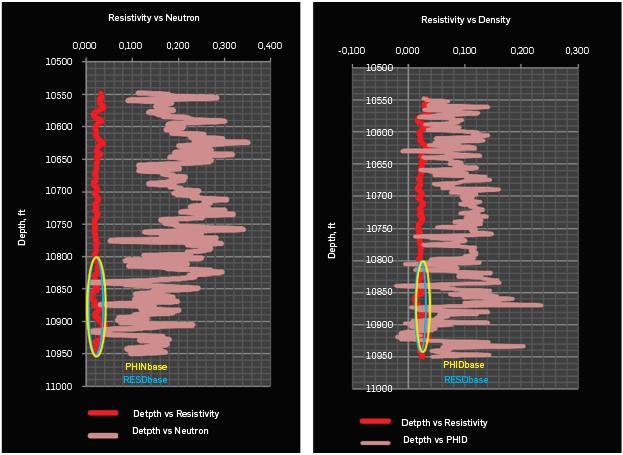Figure 4 Resistivity- Neutron, Resistivity- Density logs versus depth.

The Schmoker method is another empirical focus for determining the TOC. It has been developed to estimate the TOC quantitatively, based on the bulk density logs data , The method is based on the bulk density measure answer for the low density organic material (-1.0 g/ cm3). The method uses the Equation 17:

Where:

TOC = Total organic content, wt%

Pb = bulk density, g/cm3

This equation assumes a constant mineral composition and porosity through the formation. Although the method was developed and refined based on specific environments, it is used frequently for TOC estimation and a wide variety of Shale Formations.

With regard to this case, a triangular distribution was done on the Monte Carlo Simulation, using the estimated results in 0.10, 0.50 and 0.95 percentiles.

In terms of thickness, a triangular distribution was selected, applying the three possible minimum, more probable and maximum values (respectively 150 ft, 300 ft y 402 ft for the case study).

E.R (Ross) Crain described a twelve-step deterministic solution to reduce uncertainty in reservoirs rich in kerogen. It can be intended for hydrocarbon potential estimation in shale unconventional reservoirs. Some of the steps involved in the Crain program were adapted to a probabilistic simulation in order to verify the results obtained from the proposed procedure. The 12-step program from Crain used for validation purposes is illustrated in the Figure 5 scheme.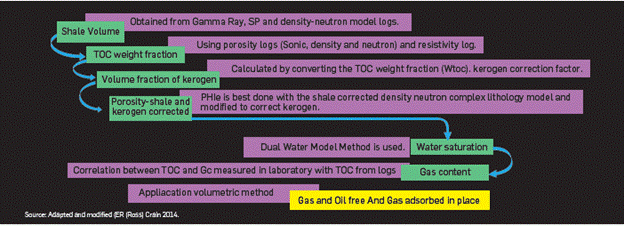Figure 5 Diagram to determine the hydrocarbon potential in shale.

Calculations of Shale volume can be obtained from Gamma Ray Log, Spontaneous Potential log, from the Density-Neutron Model among others. To obtain a shale volume, at least three values were considered, and then the average value was obtained as follows:

Where:

GRsh = shale volume value from Gamma Ray, fraction

NDsh = shale volume value from Neutron-Density model, fraction

SPsh = shale volume value from SP, fraction

Vsh = shale volume, fraction

Figure 6 shows a cross-plot based on Density-Neutron model the Tablazo Formation.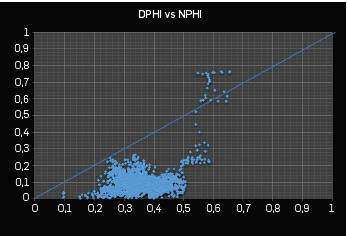Figure 6 Cross-plot indicating study zone density porosity vs neutron porosity in the Tablazo Formation.

The Crain twelve step program fundamental equations can be consulted in detail in its Petrophysical manual.

4. RESULTS ANALYSIS

In the studied case, in according to the behavior of each variable involved in the volumetric equations, the distribution assumes the normal probability for porosity, water saturation, bulk density, and gas volumetric factor, and a triangular probability distribution for the net thickness in the interest zone, the KG11, LOM (organic maturity Level) and the oil volumetric factor Bo. Check Table2 and Table 3 and the results of the probability distributions applied in some of the parameters.

Table 2 Data required for applying the distribution of normal probability to the effective porosity and the water saturation using Monte Carlo.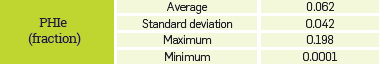Table 3 Data required for applying triangular probability distribution to the thickness using Monte Carlo simulation.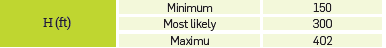According to Ribón , applying the distribution of normal probability requires average value, standard deviation, maximum value and minimum value from the data group respective to each variable; on the other hand, the triangular probability requires the minimum value, more probable, and maximum from the data group respective to each variable.

The results obtained in the simulation can be observed in the chart for the effective porosity case and the thickness formation, check Figure 7 and 8.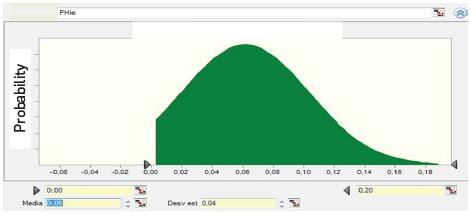Figure 7 Normal distribution for the effective porosity PHIe ARI.

Table 4 presents a comparison between the best estimated results from the procedure developed and the Crain validation for effective porosity proprieties, water saturation, and TOC% at the time to apply the respective equations and probabilities.

Table 4 Effective porosity results, water saturation and total organic content (TOC) from the procedure developed and the Crain validation applying Monte Carlo.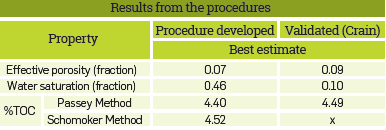Once the variable inside the properties is calculated, the volumetric equations are solved for the free gas, free oil and adsorbed gas. In the procedure developed the adsorbed gas was obtained from the total organic carbon content results (TOC) obtained from the Passey model and the Schmoker method. The data indicated in the prospective hydrocarbon resources would be for the Oil in place in the range of 51 630.3 to 51 737.8 Bls/acre and for the Total Gas in place in the range of 216.9 to 217.7 Mcf/acre. The validation using the 12-step program E.R (Ross) Crain and applying the respective probabilistic distributions obtained an adsorbed gas value of 40.57 Mcf/acre. This value is similar to that indicated before through the procedure developed, while the best estimate values of free gas 504.08 Mcf/acre and oil in place 146933.22 Bls/acre are higher. The difference is derived from the porosity values and water saturation applying E. R. (Ross) Crain, which are different than those using the proposed procedure. (Table 5).

Table 5 Free Gas results, Adsorbed Gas, Total Gas and Oil in place obtained from the procedure developed and the Crain validation applying Monte Carlo.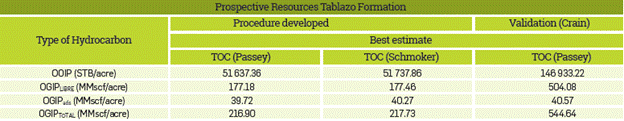The reported data in the literature concerning the amounts in Table 5, according the Sintana Energy Company, indicate that the Tablazo formation had original oil in place of 40 000 to 80 000 Bis/acre, while according to the EIA , the concentration of the Tablazo gas in place is approximately 234.8 Mcf/acre. Hence, the results were validated for Oil with the developed procedure, while the Gas results are far away from the stipulated EIA range. Furthermore, the reservoir quality and the hydraulic fluid unities for the study zone were also determined quantitatively. The petrophysical models to consider are the Kozeny-Carman and the Amafuele solutions. (Figure 9).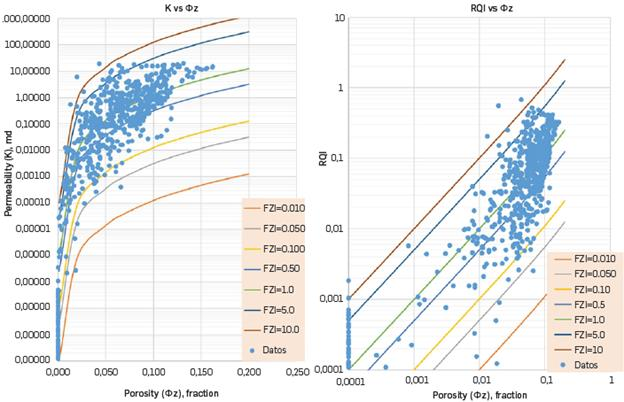Figure 9 Permeability and Reservoir Quality Index prediction vs Normalized porosity index from log data.

Another important input of this work is the brittleness estimation based on the shale fraction from the well logs, without a need for special geomechanical tests on rock samples, which is a big help in the exploration stage, where usually it is not possible to obtain data from subsurface samples; the correlation from the shale fraction (XSH) proposed is:

Where:

Xq = quartz fraction

Xc = Limestone fraction

Xd = dolomite fraction

XSH = shale fraction

Figure 10 shows the Brittleness, with 40 to 60% results suggesting good potential to develop the area, which can be compared to developing shale reservoirs such as the Barnett Shale.

CONCLUSIONS

According to the methodology set in this work, the best oil in place estimation for the Tablazo Formation in the Middle Magdalena Valley amounts to 51 637.35 to 51 737.85 Bls/acre and using the Crain validation, the best oil in place estimation would be between 146 933.00 Bls/acre. The oil estimate value by the Crain method is out of the range, trebling the oil amount. The porosity affects the volumetric estimation substantially.

A value obtained from the best effective porosity is 6.51% and according to the Crain validation 9.77%. The effective porosity value by Sintana Energy is 8% and other reports show a range of 4 to 12%. This difference between the two porosity values obtained from the two procedures evidence how a slight variation in porosity affects directly the volumetric results, inferring that the prospective resources can be overestimated.

The EIA reports a TOC range of 5,5 to 7%. With the application of the procedure developed, the best TOC estimated by the Passey method is 4.40% and by the Schmoker technic 4.51%. The Crain validation applying Passey resulted in a better estimate of 4.48%. The values obtained with the two procedures applying both techniques are found approximately in a similar range supplied by the literature. The TOC in the rock is the gas source and it also takes its own space. Excellent quality data and a good logs interpretation are necessary to obtain good estimations.

The Schmoker method to calculate the total organic content (TOC) shows a value of 4.5% leading to a better adsorbed gas estimate of 40.27 Mcf/acre. Comparing the results with that of the Passey model (for the combination of the Resistivity-Neutron the TOC), it is estimated as equal to 4.4% and a better-adsorbed gas estimate of 39.72 Mcf/acre. The Passey methodology average results from the combination of the Resistivity-Density and Resistivity-Neutron logs the TOC is 4.49% for the respective better-adsorbed gas estimate of 40.56 Mcf/acre. The higher the organic content calculated, the higher the estimate of adsorbed gas in place.

The best estimate water saturation value (M. ARI) from the proposed procedure is 0.45 and by the Crain validation (M. Simandoux) 0.10. Water Saturation values reported by the literature for the Tablazo formation are below 0.58. Water saturation is a further key parameter with direct influence on the volumetric application as the higher the water saturation, the lower the amount of hydrocarbon saturation. In the Crain validation, the volumetric calculation of Total Gas and Oil in place best estimates are OOIP 146933.22 Bls/acre and OGIP Total of 549.38 Mcf/acre.

ACKNOWLEDGEMENTS

The authors thank Escuela de Petróleos at Universidad Industrial de Santander, SPS-oil Company and Instituto Colombiano del Petróleo (ICP) for the support provided, which enabled this work

REFERENCES

 Crain. (2015). Petrophysical Handbook. [Online] E.R (Ross) Crain. Shareware Petrophysics Training and Reference Manual. [Cited March 10, 2015]. Available at: JRL Available at: JRL http://spec2000.net/01-index.htm . [ Links ]

 Crain E.R Ross (2010). Jnicorns In The Garden Of Good And Evil: Part 1- Total Organic Carbon (TOC). [ Links ]

 ORACLE, "Oracle Crystal Ball", 2015. Available: http://www.oracle.com/technetwork/middleware/crystalball/overview/index.html. [ Links ]

 Vargas, C. (2012). Evaluating total yet-to- find hydrocarbon volumen in Colombia. Earth Sciences Research Journal, Volume 16, Special Issue, Universidad Nacional de Colombia: Research Group in Geophysics. [ Links ]

 Crain, E.R Ross. (2014) HOLGATE D. Step Program To Reduce Uncertainty In Kerogen-Rich Reservoirs: Part 1- Getting the right porosity. Reservoir Issue 03. [ Links ]

 Passey, Q.R; et al. (2010). From Oil-Prone Source Rock to Gas-Producing Shale Reservoir-Geologic and Petrophysical Characterization of Unconventional Shale-Gas Reservoir. Available at: Society of Petroleum Engineers, SPE 131350/SPE International, Copyright 2010. 29p. [ Links ]

 Charsky, A. and Herron, S. (2013). Accurate, Direct Total Organic Carbon (TOC) Log from a New Advanced Geochemical Spectroscopy Tool: Comparison with Conventional Approaches for TOC Estimation Search and Discovery Article # 41162. [ Links ]

 Ribón, H. (2012). Cuantificación de Recursos Hidrocarburíferos prospectivos en un sector de una cuenca frontera. Caso de aplicación: cuenca fronteracolombiana. M.Sc. thesis, Petroleum Engineering School. Universidad Industrial de Santander. Colombia. 2012. [ Links ]

 US. EIA. (2013). Technically Recoverable Shale Oil and Shale Gas Resources: An Assessment of 137 Shale Formations in Countries outside the United States. Available at: https://www.eia.gov/analysis/studies/worldshalegas/pdf/overview.pdf. [ Links ]

 Schmoker, J., and T. Hester. (1983) Organic Carbon in Bakken Formation, United States Portion of Williston Basin, American Association of Petroleum Geologists Bulletin, 67, 2165-2174. [ Links ]

Recebido: 26 de Janeiro de 2016; Revisado: 01 de Outubro de 2018; Aceito: 14 de Novembro de 2018

* email: ludyamparoguto@gmail.comThis is an open-access article distributed under the terms of the Creative Commons Attribution License